Home

 << Previous    1...   2  3    5  6  ...24    Next >>

4. The sacred-geometrical character of the 24-cell

The 24 vertices of each of the (5+5) 24-cells making up the compound of two 600-cells whose 240 vertices determine the 240 root vectors of E8 denote gauge charges of this Yang-Mills gauge symmetry group. These charges are spread along each of the (5+5) half-revolutions of all 10 whorls of the UPA as the subquark state of the E8×E8 heterotic superstring. The 24-cell is unique amongst n-polytopes (n≥4) in being the only one other than the n-simplex that is self-dual. In fact, it is holistic, too, for the following reason: the 24-cell has 24 vertices, 96 edges, 96 triangular faces & 24 octahedral cells. Its 0-, 1-, 2- & 3-polytopes comprise 120 vertices & edges and 120 faces & cells. This 120:120 division of its 240 polytopes is characteristic of holistic systems, as, of course, is its embodiment of the holistic parameter 240. It is a repetition of the archetypal pattern discussed earlier in the compound of two 600-cells, each with 120 vertices, and in other sacred geometries previously compared with them. Its polytope composition conforms to the hexagonal yod composition of the seven regular polygons making up the inner form of the Tree of Life, as shown below: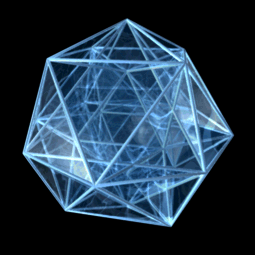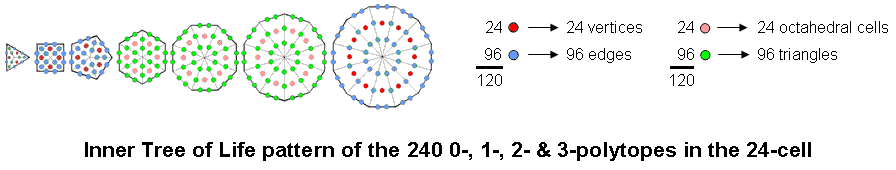The 24-cell consists of 240 polytopes: 24 vertices;96 edges;96 triangles;24 octahedral cells. Constructed from tetractyses, the 7 separate polygons of the inner Tree of Life have 240 hexagonal yods:The triangle, square, pentagon & dodecagon have 120 hexagonal yods. They comprise 24 dark red hexagonal yods at the centres of 24 tetractyses and 96 blue hexagonal yods on their sides. The former correspond to the 24 vertices of the 24-cell and the latter correspond to its 96 edges. The hexagon, octagon & decagon have 120 hexagonal yods. They comprise 24 light red hexagonal yods at centres of tetractyses and 96 green hexagonal yods on their sides. The former correspond to the 24 octahedral cells in the 24-cell and the latter correspond to its 96 triangular faces.

(See also the discussion on #1 (see under heading "24-cell") of other properties of the 24-cell that indicate its holistic character, e.g., the 384 sides of the simple triangles in its faces when they are Type A). Further evidence that the 24-cell has a holistic character is the fact that the 120:120 division of its polytopes mirrors the 120:120 division of the 240 geometrical elements surrounding the centres of the two Type B dodecagons in the inner Tree of Life, each dodecagon corresponding to half of the 24-cell: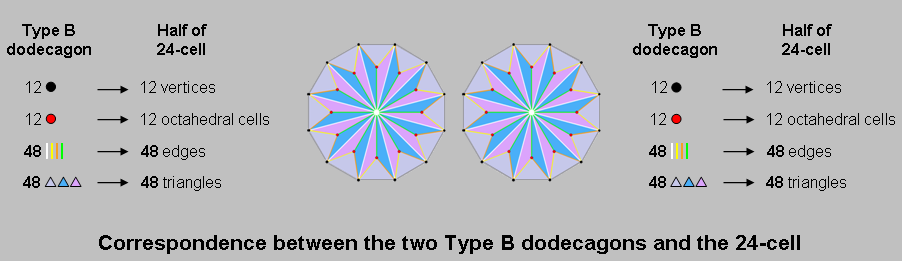Their 24 black corners correspond to the 24 vertices of the 24-cell, the 24 red corners of their 72 triangles correspond to its 24 octahedral cells, the 96 interior sides of their triangles correspond to its 96 edges and the 96 sides & triangles correspond to its 96 triangles. The same 24:96:96:24 pattern is found in the 240 boundary yods of the two separate sets of seven enfolded polygons, each set representing half the 24-cell: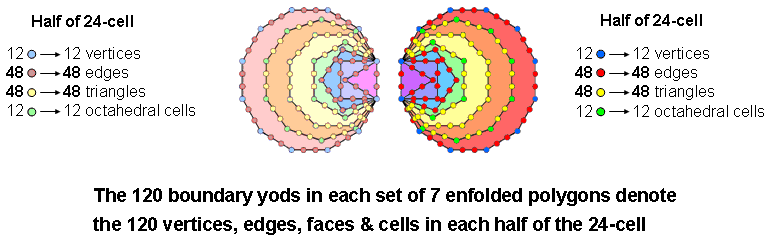It also occurs in the 240 hexagonal yods in the faces of the tetrahedron, octahedron & cube when they are constructed from tetractyses: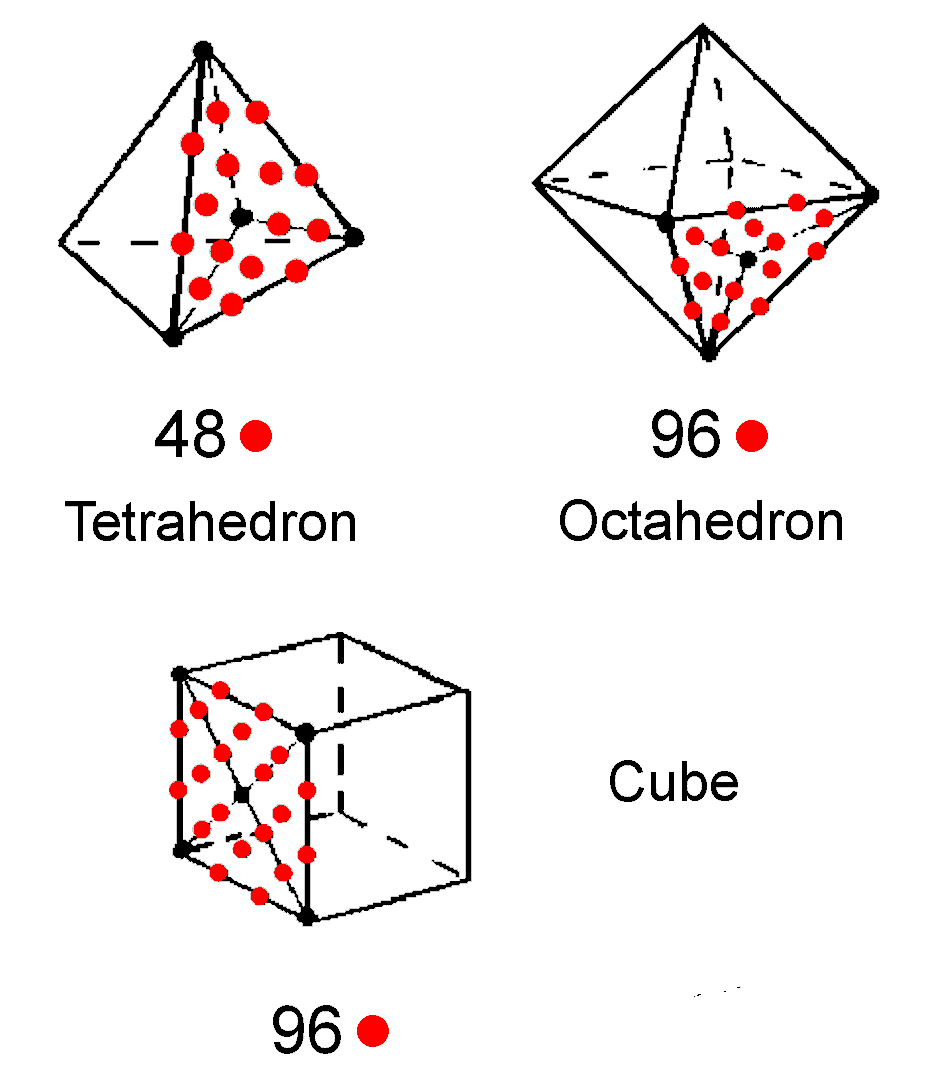Platonic solid Edges Faces Hexagonal yods at centres Hexagonal yods on edges Hexagonal yods on interior sides Total Tetrahedron 6 4 4×3 = 12 6×2 = 12 4×6 = 24 48 Octahedron 12 8 8×3 = 24 12×2 = 24 8×6 = 48 96 Cube 12 6 6×4 = 24 12×2 = 24 6×8 = 48 96 Total 30 18 60 60 120 240

The 120 hexagonal yods either at their centres or on their edges comprise (12+12=24) in the tetrahedron and (24+24+24+24=96) in the octahedron & cube (indicated in the red cells); the 120 hexagonal yods lining interior sides of tetractyses in their faces comprise 24 in the tetrahedron and (48+48=96) in the octahedron & cube (shown in the blue cells). It cannot be accidental that there is such repetition of this pattern in objects like the inner Tree of Life, the dodecagon and the Platonic solids — objects which have been previously established as possessing sacred geometry because they exhibit its defining parameters and display the same patterns that relate them. Rather, its re-occurrence in the 24-cell points towards the holistic character of this polychoron and confirms its connection to both superstring physics and the structure of the UPA.

As

24 = 52 − 1 = 3 + 5 + 7 + 9

is the sum of the first four odd integers after 1 and

120 = 112 − 1 = 3 + 5 + 7 + 9 + 11 + 13 + 15 + 17 + 19 + 21,

is the sum of the first 10 integers after 1, we see that the Pythagorean Tetrad and Decad determine both numbers and their difference:

96 = 120 − 24 = 11 + 13 + 15 + 17 + 19 + 21.

The 24 vertices (or octahedral cells) and 96 edges (or triangles) possessed by the 24-cell follows the arithmetic pattern of the first four and the last six odd integers in the first 10 odd integers! This 4:6 division parallels the prediction by superstring theory that space-time has 10 dimensions, of which four form the large-scale Minkowski space-time continuum and six are compactified.

The question next to be asked is: given that a 24-cell determines 24 E8 gauge charges that are spread along a half-revolution of all 10 whorls of the UPA/E8×E8 heterotic superstring around its axis of spin, i.e., 1680 turns of these helices, how does the polychoron generate this winding number as a component of the 10 24-cells whose 240 vertices define the roots of E8? Each charge is spread uniformly over 70 turns in a half-revolution of all 10 whorls, seven per whorl, so that the three major whorls have 21 such turns and the seven minor whorls have 49 such turns. This number is highly significant vis-à-vis the Tree of Life because its 16 triangles have 70 yods when they are tetractyses (see here). This identification is consistent with the 2nd-order tetractys and the distinction between the three major and seven minor whorls of the UPA because the (21+49=70) hexagonal yods in the (3+7=10) 1st-order tetractyses of the 2nd-order tetractys consist of 10 sets of seven hexagonal yods. It would represent an E8 gauge charge, the 70 yods denoting 70 turns in 10 whorls, each 1st-order tetractys denoting a half-revolution of a single whorl and the seven hexagonal yods in each 1st-order tetractys symbolising the seven turns per whorl: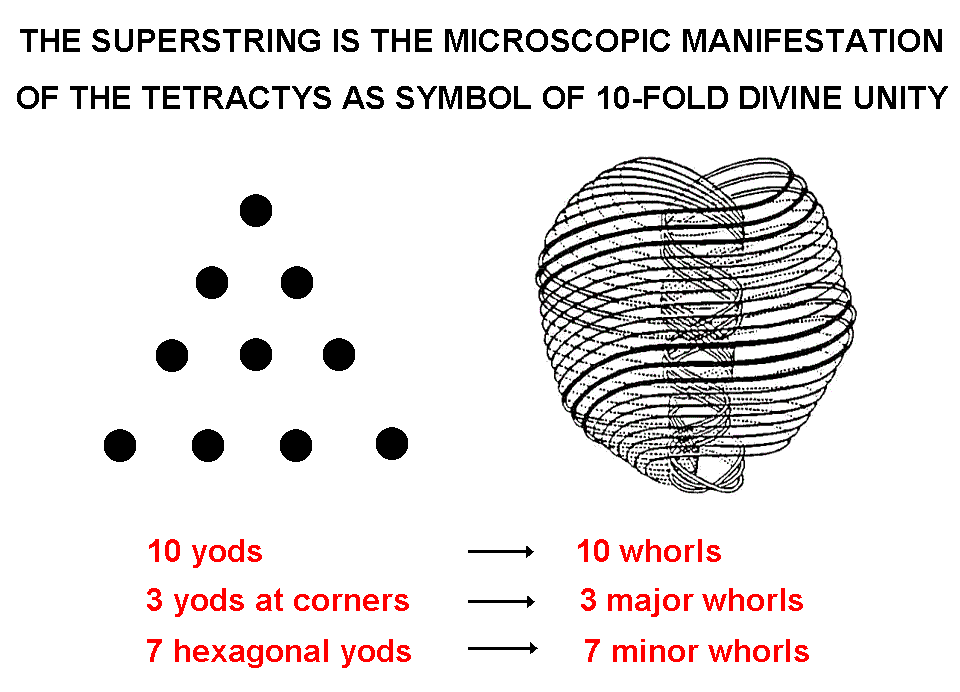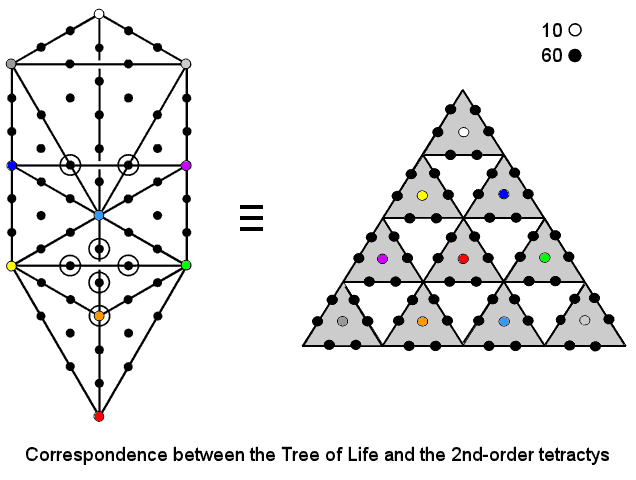It is well-known that the chromatic number for a graph drawn on a 2-torus T2 is 7. The polychorons have an Euler characteristic of 0. This happens to be the same as the Euler characteristic of an n-dimensional torus Tn, which is the product space of n circles. This is precisely how Leadbeater described the six orders of circular turns higher than the ones making up each whorl of the UPA. Given that the 240 E8 gauge charges are the sources of 10-dimensional gauge fields, it is as though they are spread along the 10 whorls, 120 in each inner or outer half corresponding to the 120 vertices of each 600-cell, and that each whorl (or, rather, seven turns in a whorl) generates a space-time component of each gauge field:

16800 turns in 10 whorls ≡ 240 E8 gauge charges × 10 space-time components of E8 gauge field ×7.

7 turns ≡ space-time component of E8 gauge field.

This, however, is still a speculation that needs to be proved or disproved because the mathematical connection between this chromatic number and the 10-fold subquark state of the E8×E8 heterotic superstring needs yet to be established. But it is clear that sections of seven turns in each whorl have holistic significance, for C.W. Leadbeater stated that every circular turn was comprised of seven coils that wound around a smaller circle, and that each coil was a helix made up of seven still smaller coils, and so on. This means that the six higher orders of what he called "spirillae" (see here) represent the winding of a curve around six successively smaller circles, all mutually orthogonal, i.e., the six compactified, circular dimensions of the 6-torus T6. Whatever is responsible for a 7-fold winding around each compactified dimension (obviously connected to the chromatic number 7 for a 2-torus) is responsible for an analogous winding around every seven circles making up a whorl wound on a 2-torus. It is highly significant that he should have counted seven coils of the next higher order making up a coil of a given order in a minor whorl. This establishes a connection between his counted number (1680) of turns in a whorl and the group-theoretical number 240 of roots in the Lie group E8:

1680 = 240×7.

It indicates that, indeed, sections of seven turns — to whatever order of spirilla they belong — do represent physically significant things, in this case (if the above interpretation is correct) the space-time component of a gauge field of E8. The question that needs answering is why the 168 helical turns in each half-revolution of a whorl are the string manifestation of 24 E8 gauge charges. It is like the question: why are there 24 hours in a day and a week of seven days with 168 hours? Is the re-appearance of these numbers no more than a coincidence? Or are they a reflection of the existence of a fundamental cosmic cycle that happened to be reproduced in ancient times when, firstly, the ancient Egyptians used the practice in the earlier Sumerian culture of counting with their thumb against the three knuckles of the other four fingers to divide the day (and then the night) into 12 parts that only became equal in ancient Greece under the influence of its great astronomer Hipparchus and, secondly, the creation myths of Judaism and ancient Babylonian religions gave rise to a seven-day week based upon seven stages of creation? If the UPA exists as 10 whorls because superstring space-time is 10-dimensional, then its three major whorls bear a formal correspondence to the three large-scale dimensions and its seven minor whorls correspond to the six compactified dimensions and the dimension of time, so that the breakdown in the unified symmetry of E8 arising from the difference of scale between these two classes of dimensions led to a difference between three whorls that generate the three components of the gauge fields in large-scale space and the remaining seven whorls. This is what Leadbeater noticed in the UPA, for three whorls, which he called "major," were thicker than the seven other "minor" ones (see here for the reason for this difference). The difference, of course, in Kabbalistic terms is that between the Supernal Triad of Kether, Chokmah & Binah and the seven Sephiroth of Construction. In Pythagorean terms, it is the difference between the yods at the three corners of the tetractys and its seven hexagonal yods (see here).

Shown below are the Petrie polygons of the 24-cell, the 600-cell and the 421 polytope: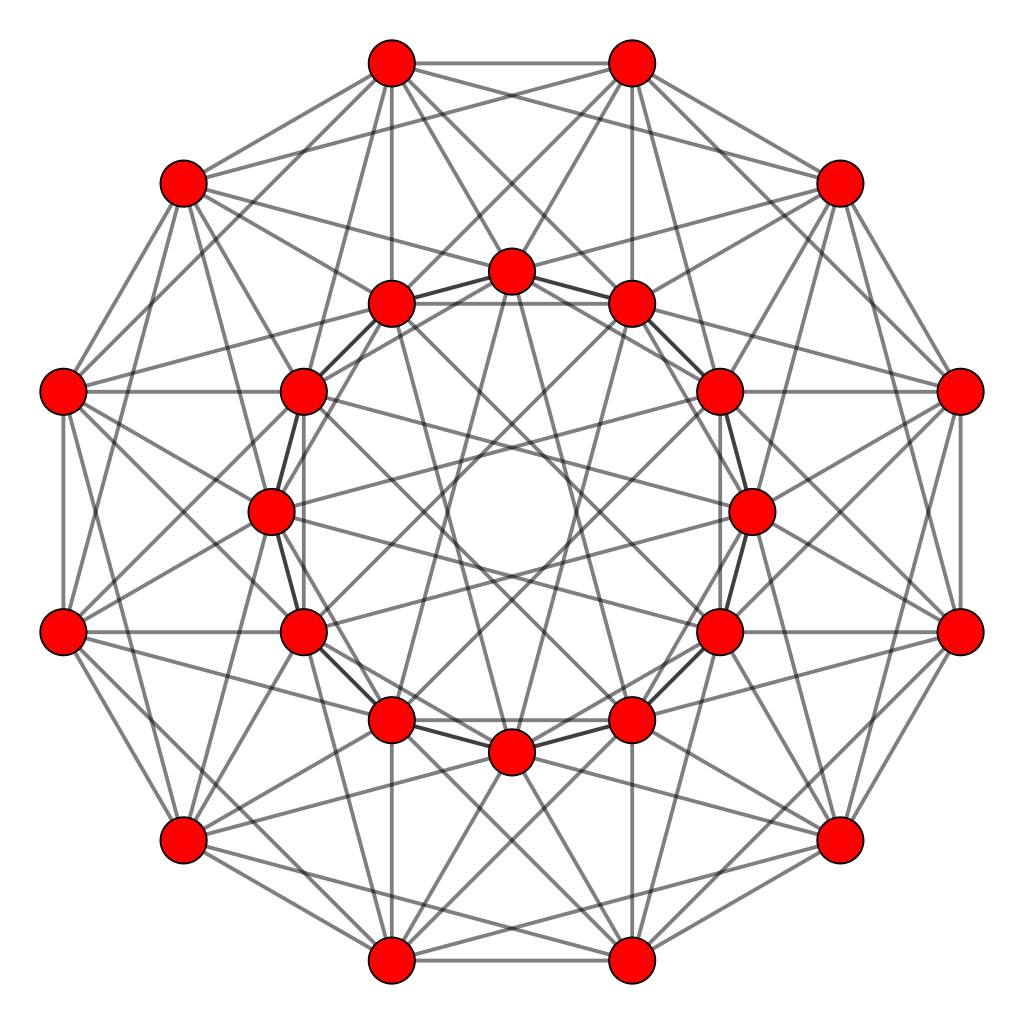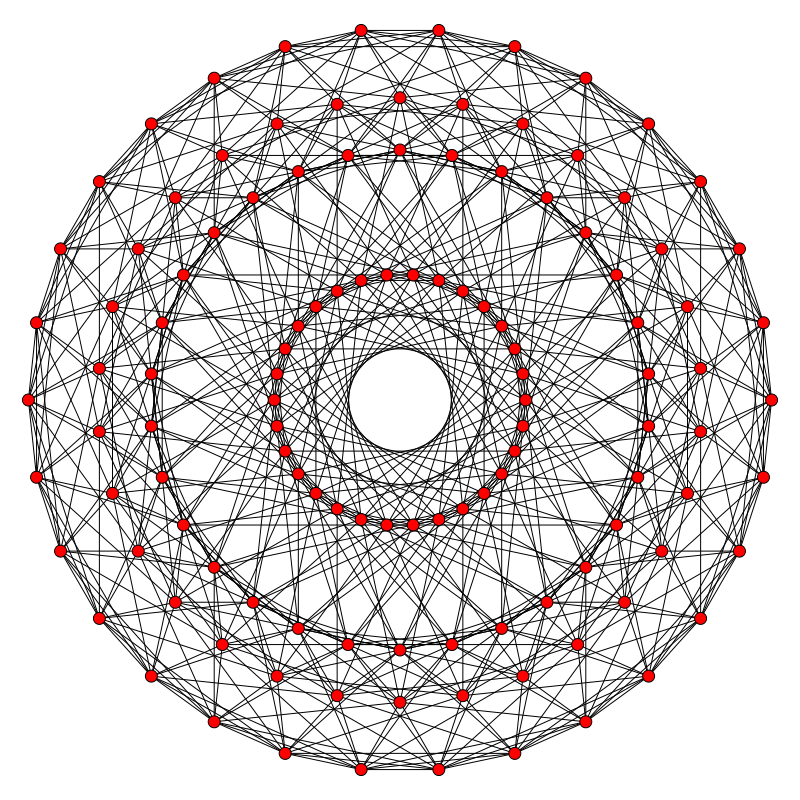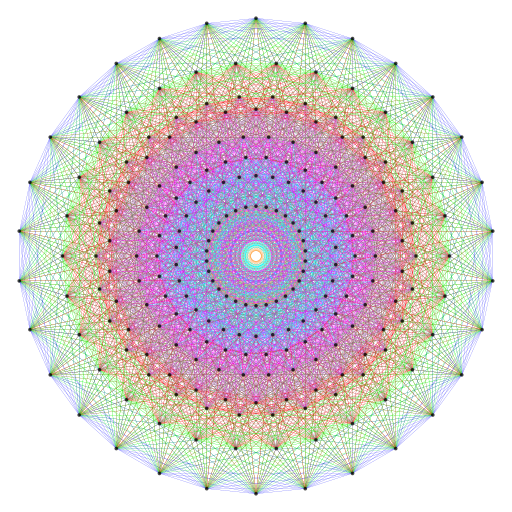Petrie polygon of the 24-cell is the dodecagon Petrie polygon of the 600-cell is the triacontagon Petrie polygon of the 421 polytope is the triacontagon (To enlarge, place mouse pointer over image) ×5 → ×2 →

The Petrie polygon of the 24-cell is the dodecagon, which is the last of the seven regular polygons making up the inner Tree of Life. The Petrie polygon of the 600-cell, a compound of five 24-cells, is the regular 30-gon, as is the Petrie polygon of the 421 polytope because it is a compound of two concentric 600-cells of different size but with the same orientation. As 30/12 = 2½, the factor of 5 between the 120 vertices of the 600-cell and the 24 vertices of the 24-cell manifests in the repetition of the outermost two circular rows of 30 vertices in the Petrie polygon of the former. The factor of 10 between the 24 vertices of the 24-cell and the 240 vertices of the 421 polytope arises from the repetition of the four outermost circular rows of 30 vertices in the four innermost circles. Remarkably, comparison of the Petrie polygons of the 24-cell and the 421 polytope reveals the same ×10 and ×2½ factors as those manifesting in the UPA, namely, the 10 half-revolutions of its whorls (or the 10 whorls) and the 2½ revolutions in its inner or outer halves. For the unbelieving sceptic, this has to be dismissed as just another coincidence, along with the (840+840=1680) vertices & edges of the two 600-cells, whose embodiment of the structural parameter 1680 can be for such a person no more than the product of chance. However, for those who do not believe in miracles, the presence of the factors 2½, 5 & 10 in the Petrie polygon of the 600-cell makes the embodiment in its geometry of the UPA structural parameter 840 all the more significant, providing yet more compelling evidence that this particle remote-viewed over a century ago is the E8×E8 heterotic superstring. At the same time, it must be pointed out that the 421 polytope occupies 8-dimensional space, whereas the features of the UPA being compared to it or to its 4-dimensional, Coxeter plane projection pertain to 3-dimensional space. Even though the two spaces are different, it should not be hard to accept that the features that pertain to the space occupied by a polychoron representation of the E8 roots/gauge charges carried by a superstring should also apply to their manifestation in the 4-dimensional cross-section of 10-dimensional space-time.

Here is another reason why we should take seriously these correspondences between mathematical facts, sacred geometrical patterns and alleged paranormal descriptions of the basic unit of matter. The dodecagon needs 168 more yods to transform its 12 sectors into Type A triangles, turning into a Type B dodecagon: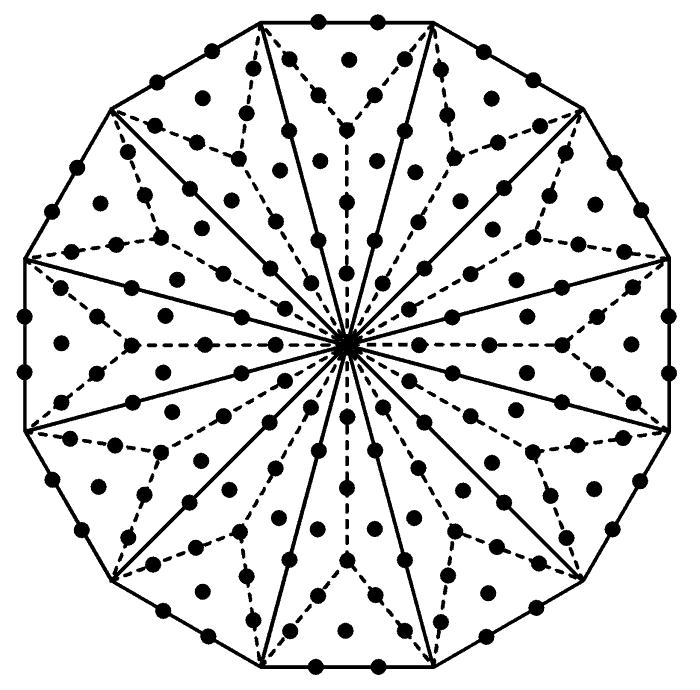Notice that this regular polygon is the Petrie polygon of the 24-cell. Might we not expect, therefore, that the 24-cell has a connection to the number 1680 — the number of turns in a half-revolution of the 10 helical whorls of the UPA that, according to our earlier analysis, "carries" 24 E8 gauge charges denoted by the vertices of this polychoron? Yes, as now explained. Taking the edge length of the 24-cell as 1, eight of its vertices have coordinates that are all the permutations of (±1, 0, 0, 0); they are vertices of the 16-cell: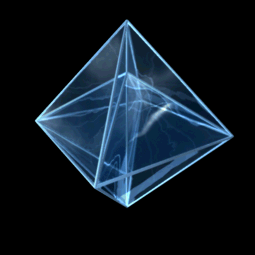Rotating 16-cell.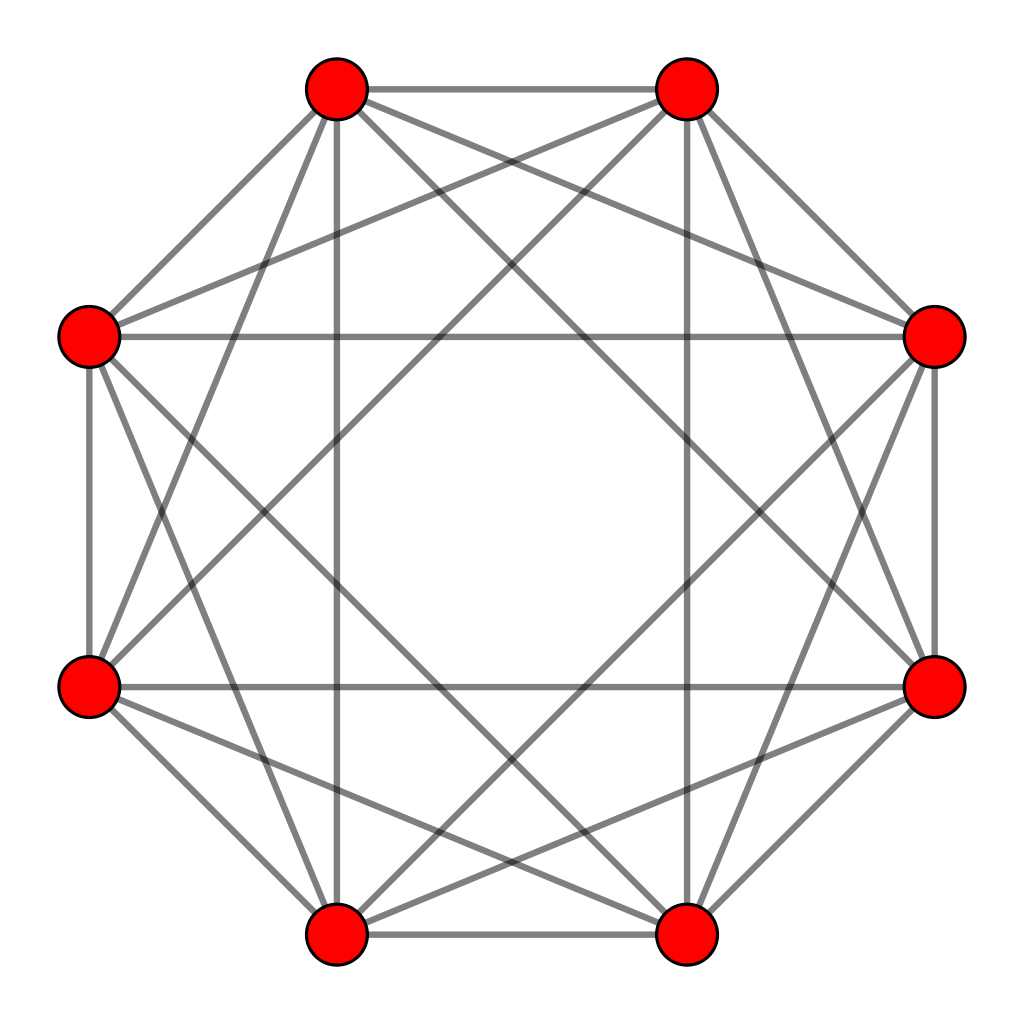Petrie polygon of the 16-cell. Isometric Orthogonal Projection of: 8-Cell (Tesseract) + 16-Cell = 24-Cell Credit: Title: Cell24Construction.ogv Author: Jgmoxness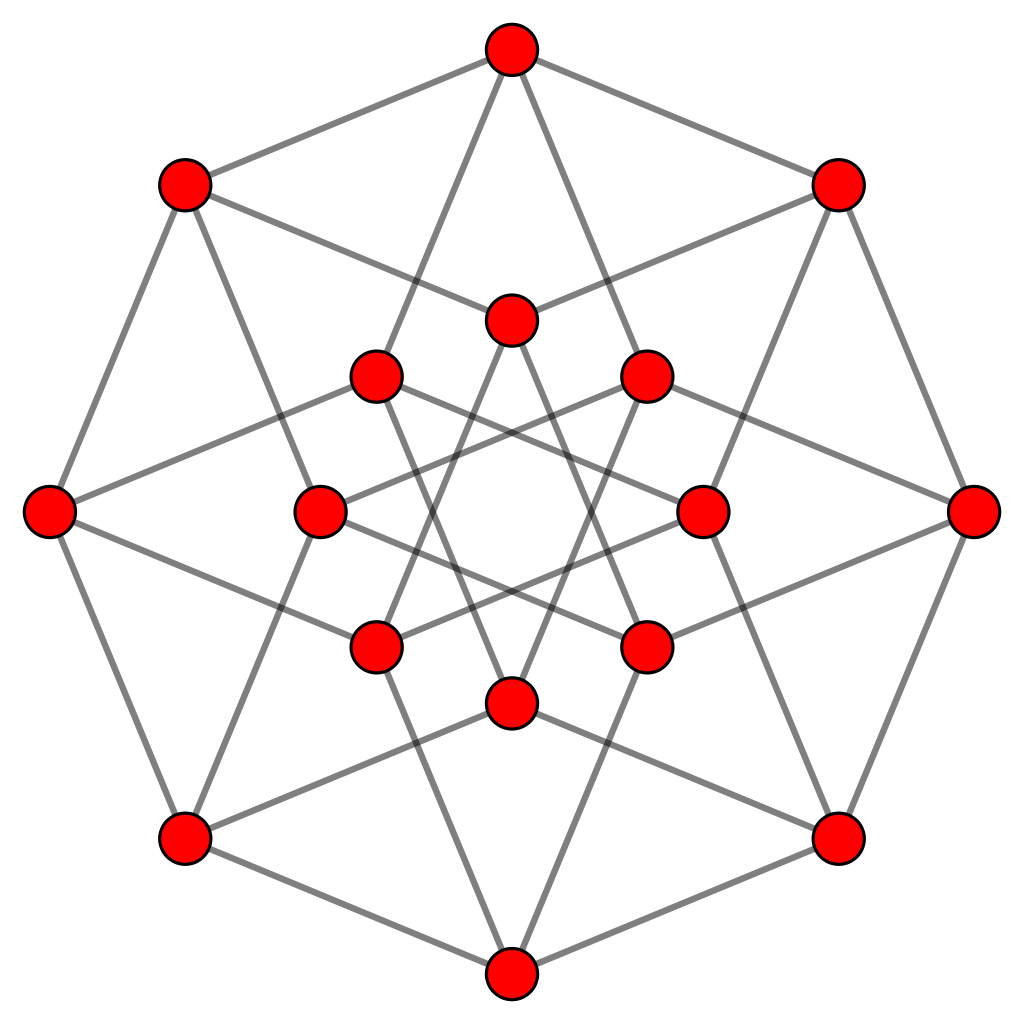Rotating 8-cell. Petrie polygon of the 8-cell.

16 vertices of the 24-cell are of the form: (±½, ±½, ±½, ±½); they are the vertices of an 8-cell. They can be divided into two groups of eight: those with an even number of (−) signs and those with an odd number of (−) signs. Each group of vertices defines a 16-cell. Therefore, the 24 vertices of a 24-cell can be grouped into three sets of eight, each set defining a regular 16-cell. According to Table 2 on #1, the 16-cell is composed of 168 triangles when its faces and interior are constructed from Type A triangles, whilst the 24-cell comprises 576 triangles, where 576 = 242. Here, therefore, is a mathematical connection between the superstring structural parameter 168 and the 24-cell. According to comment (1) about the 8-cell on #1, 168 points & lines compose its 24 square faces. This is analogous to the 1680 vertices & edges making up the 2400 faces in the compound of two 600-cells that is the Petrie projection of the 421 polytope. We see that this number (the gematria number value of Cholem Yesodeth, the Mundane Chakra of Malkuth) manifests as well in both the 8-cell and the 16-cell that make up each of the 10 24-cells in this compound. In view of this, it should come as no surprise that the 24-cell plays a central role in the complete, superstring explanation of the 10 whorls of the UPA ....

The 24-cell constructed from Type A triangles
We saw earlier that the 240 hexagonal yods in the seven regular polygons making up the inner Tree of Life symbolise the 240 0-, 1-, 2- & 3-polytopes in the 24-cell. Additional confirmation of its holistic character is provided by its construction from Type A triangles: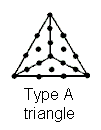The 24-cell has:

24 vertices
96 edges
96 triangular faces
24 octahedra

Numbers of geometrical elements other than vertices & edges surrounding centre of 24-cell

 Corners Sides Triangles Total Faces 96 3×96=288 3×96=288 672 Interior 96 24 + 3×96 = 312 3×96=288 696 Total 192 600 576 1368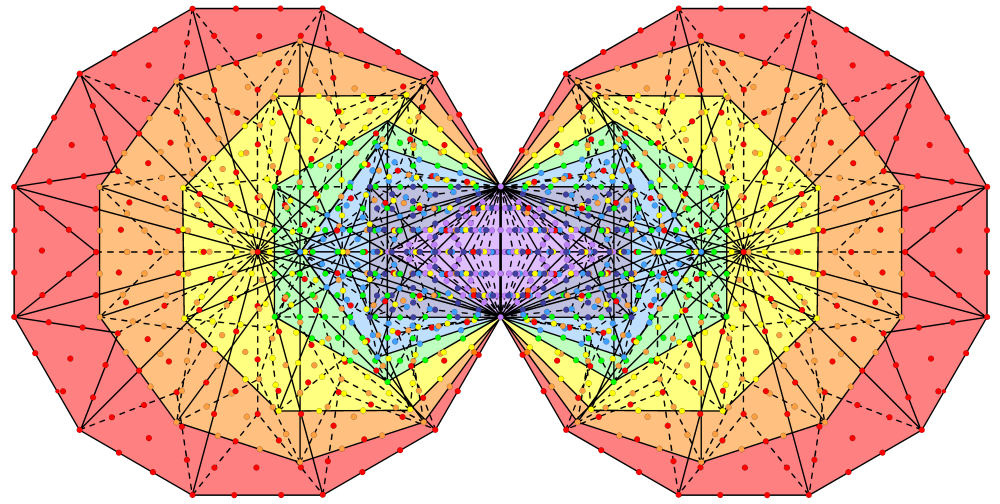Rotating 24-cell    1368 yods are intrinsic to the (7+7) Type B polygons enfolded in each overlapping Tree of Life

When the 96 internal triangles formed by its edges and its 96 faces are Type A triangles, the centre of the 24-cell is surrounded by 1368 corners, sides & triangles other than its 24 vertices and 96 edges. In other words, given the latter, 1368 more geometrical elements are needed to construct the polytope. The inner form of the Tree of Life with Type B polygons contains 1370 yods. Of these, the topmost corners of the two hexagons coincide with the lowest corners of the hexagons enfolded in the next higher Tree of Life. This means that 1368 yods are intrinsic to each set of 14 enfolded Type B polygons. They denote the 1368 extra geometrical elements in the 24-cell built both internally and externally out of Type A triangles. It demonstrates that this polytope conforms to the holistic pattern of the inner Tree of Life. This is true also when the seven regular polygons are separate, for the 672 extra geometrical elements in its faces comprise 96 corners, 288 sides & 288 triangles, and these numbers are the extra corners, sides & triangles added when the (7+7) separate Type A polygons are turned into Type B polygons:

corners: 48 + 48 = 96;
sides: 3×48 + 3×48 = 288;
triangles: 3×48 + 3×48 = 288.

Total = 672.

Each half of the 24-cell has 336 extra geometrical elements. There are (5×672=3360) extra geometrical elements in the five 24-cells (1680 in each half), the compound of which is the 600-cell. The presence of the three UPA structural parameters 336, 1680 & 3360 in the geometry of the 600-cell is strong confirmation that the UPA is an E8×E8 heterotic superstring. There are 6720 extra geometrical elements in the 10 24-cells making up the compound of two 600-cells. Compare this with the 3360 circularly polarised waves in each revolution of the 10 whorls of the UPA. Each wave is a supposition of two plane waves with a phase difference of 90°. Therefore, 6720 plane waves circulate once around the axis of the particle. It is hard to resist the conclusion that they are the manifestation of the 6720 extra geometrical elements required to construct the 10 24-cells from Type B triangles. This is particularly so given that the 421 polytope has 60480 faces with 240 vertices and 6720 edges! In other words, the very 8-dimensional polytope whose vertices determine the 240 root vectors of E8 has exactly the same number of edges as:

• the number of orthogonal plane waves that generate the 3360 circularly polarised oscillations in one revolution of the 10 whorls of the UPA about its axis of spin;
• the number of geometrical elements generated by dividing into their sectors the faces of the 10 24-cells in the compound of two 600-cells that is the 4-dimensional, Coxeter plane projection of the 421 polytope.

Given that 672 extra geometrical elements are needed to divide the 96 faces of the 24-cell into their 288 sectors, is it a coincidence that, as shown here, 672 yods are needed to construct the first four Platonic solids from Type A triangles? Why should the 421 polytope, which mathematicians know reproduces the 240 root vectors of the exceptional Lie group E8 present in one of the two heterotic superstring theories, have exactly ten times as many edges as these Platonic solids contain yods when they are constructed from Type A triangles? Why, as pointed out on #2, should the number of yods (13680) lining the edges of the faces of this polytope when they are tetractyses be exactly ten times the number of yods that are intrinsic to the inner form of one Tree of Life with Type B polygons? Why should this number be exactly the number of yods intrinsic to the inner form of ten overlapping Trees of Life? Either miraculous coincidences are in play here or we are witnessing the remarkable appearance in different systems of geometry of the same number simply because all these systems possess sacred geometry characterised by the same parameters (apart from a Pythagorean factor of 10 in some cases). The reader must decide which is the more plausible possibility.

Inner Tree of Life pattern of the 24-cell
When the 96 faces of the 24-cell and the 96 internal triangles formed by its edges are Type A triangles, their (3×96 + 3×96 = 576) triangular sectors have (1+24+96+96=1+216=217) corners, where "1" denotes the centre of the polychoron. The number 216 of Geburah is the number of corners of triangles that surround the centre of the 24-cell. Compare this with the fact that, of the 264 yods in the seven enfolded Type A polygons with tetractyses as their 47 sectors, (264−47=217) yods line the sides of the tetractyses. Of these, one yod at the topmost corner of the hexagon coincides with the lowest corner of the hexagon enfolded in the next higher Tree, leaving 216 boundary yods that are intrinsic to the set of polygons enfolded in each Tree. The shared corner, which is unique because it also belongs to another set of polygons, corresponds to the centre of the 24-cell and the 216 boundary yods of the sectors of the seven enfolded polygons correspond to the 216 corners of the 576 sectors of the 192 Type A triangles from which the polychoron is constructed. Notice also that (1 + 24×2 = 49) yods line the 24 sides of the 96 internal triangles formed by the edges of the 24-cell, where 49 is the number of EL CHAI, the Godname of Yesod, and that (24 + 2×96 = 216) yods line the edges of the 24-cell — just as they do for the sectors of the seven enfolded polygons. This correspondence is further evidence that the 24-cell conforms to the holistic pattern of the inner Tree of Life.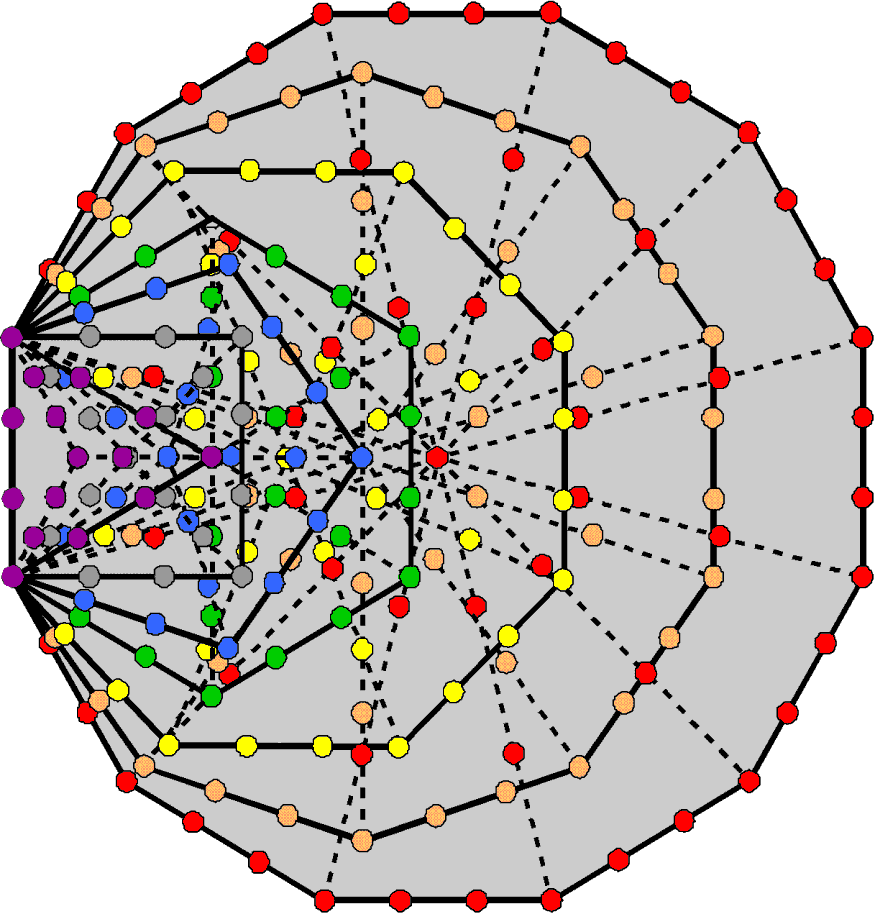Lining the 88 sides of the 47 tetractys sectors of the 7 enfolded polygons are 216 yods, excluding the topmost corner of the hexagon that is shared with the hexagon enfolded in the next higher Tree of Life. This is the number of yods on the 96 edges of the 24-cell when its faces are tetractyses. 2160 yods line the 880 sides of the 470 tetractys sectors of the 70 polygons enfolded in 10 Trees of Life. This is the number of yods on the edges of the 10 disjoint 24-cells making up the compound of two 600-cells. A 24-cell is to a 600-cell what a single Tree of Life is to 10 Trees representing its 10 Sephiroth. It indicates that the two centre-sharing 600-cells are the 4-dimensional counterparts of the two sets of five Sephiroth — or, rather, the two sets of five Trees of Life that represent them.

It suggests that we should carry out a more thorough analysis of the yod composition of the 24-cell. Tabulated below is the numbers of yods in its faces and interior:

 Face/internal triangle Faces Interior Total Corners Hexagonal yods Total Corners Hexagonal yods Total Corners Hexagonal yods Total Tetractys 24 96×2 + 96 = 288 312 1 24×2 + 96 = 144 1+144=145 1+24=25 432 1+456=457 Type A triangle 24+96=120 96×2 + 96×9 = 1056 1176 1+96=97 24×2 + 96×9 = 912 1+1008=1009 1+216=217 1968 1+2184=2185 Type B triangle 24 + 96×4 = 408 96×2 + 96×33 = 3360 3768 1 + 96×4 = 385 24×2 + 96×33 = 3216 1+3600=3601 1+792=793 6576 1+7368=7369

• Its 96 faces are composed of 312 yods when they are tetractyses. 312 is the 155th even integer after 2, the first even integer, where 155 is the number value of ADONAI MELEKH, the Godname of Malkuth:Each half of the 24-cell has 216 hexagonal yods, where 216 is the number of Geburah. There are 457 yods, of which 24 are vertices, leaving 433 generated by the transformation of the faces into tetractyses. 433 is the 84th prime number, where 84 = 12 + 32 + 52 + 72.
• 216 corners surround the centre when the faces are Type A triangles. 97 of these are internal, where 97 is the number value of Haniel, the Archangel of Netzach. The five disjoint 24-cells in the 600-cell have (1 + 5×216 = 1081) corners, where 1081 is the number value of Tiphareth ("Beauty"). Including its centre, there are 1081 yods other than vertices in either half of the 24-cell with Type A triangles as its faces.
• When the faces are Type B triangles, they have 384 corners that are not vertices, 192 in each half. The number 384 and its 192:192 division are characteristic of sacred geometries (see The holistic pattern). There are 385 corners inside the 24-cell, where 385 = 12 + 22 + 32 + 42 + 52 + 62 + 72 + 82 + 92 + 102. There are 3360 hexagonal yods in the 96 Type B faces. This means that the 600-cell contains (5×3360=16800) hexagonal yods when its five 24-cells have Type B triangles as their 480 faces. The compound of two 600-cells that is the 4-dimensional projection of the 421 polytope defining E8 has 10 24-cells with 33600 hexagonal yods in their 960 faces. This is the amazing way in which the superstring structural parameters 3360 and 16800 are embodied in the 24-cell and 600-cell, themselves holistic entities like the whorl and UPA. Their appearance is so unambiguous that it can leave no doubt that the UPA is, indeed, an E8×E8 heterotic superstring, for its 16800 circularly polarised waves (the circular 1st-order spirillae in its 10 whorls) can be resolved into 33600 plane wave components, each pair of perpendicular plane waves differing in phase by 90°. Each hexagonal yod denotes such a plane wave, confirming our earlier conclusion that the 120 vertices in each 600-cell denote the 120 E8 gauge charges spread along either the outer or the inner half of the UPA. The 1680 turns in each half-revolution of its 10 whorls comprise 3360 plane waves, each denoted by a hexagonal yod in a 24-cell, the five half-revolutions of the outer half of the UPA being generated by the five 24-cells in one 600-cell and the five half-revolutions of its inner half being generated by the five 24-cells in the other concentric 600-cell. In other words, each of the 10 24-cells in the compound of two 600-cells represents a half-revolution of the 10 whorls of the UPA, its 24 vertices signifying the 24 E8 gauge charges that are spread along the length of a half-revolution.

Tabulated below is the number of geometrical elements in the faces and interior of the 24-cell:

 Face/internal triangle Faces Interior Total Corners Sides Triangles Total Corners Sides Triangles Total Corners Sides Triangles Total Triangle 24 96 96 216 1 24 96 1+120=121 1+24=25 120 192 1+336=337 Type A triangle 24+96=120 96 + 96×3 = 384 96×3=288 792 1+96=97 24 + 96×3 = 312 96×3=288 1+ 696=697 1+216=217 696 576 1+1488=1489 Type B triangle 24 + 96×4 = 408 96 + 96×12 = 1248 96×9=864 2520 1 + 96×4 = 385 24 + 96×12 = 1176 96×9=864 1+2424=2425 1+792 2424 1728 1+4944=4945